### Choose language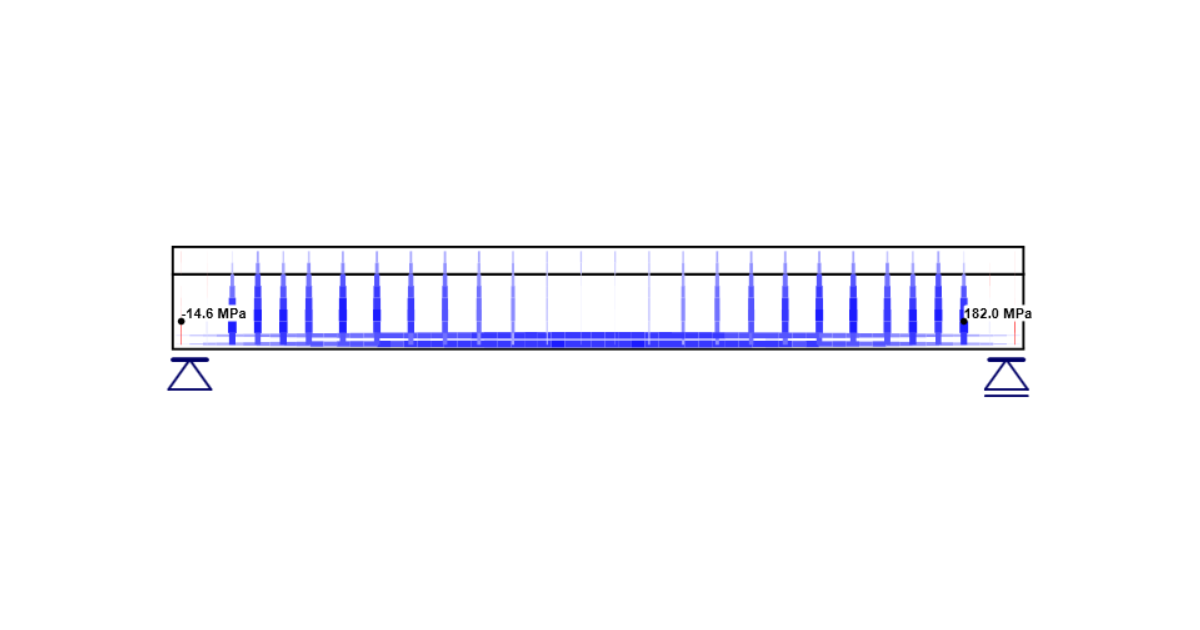# General description of ULS results in Detail application

This article dedicates to the presentation of the results in the Detail application, focusing on the Ultimate Limit State.
$$In this section, we focus on the Strength and the Anchorage (ULS checks). Notice we have a new tab (Results) in the top ribbon after choosing one of the ULS checks. On the left side, you can choose if you want to display results for an automatic extreme, a particular ULS combination, or increments. Remember that non-linear calculation is used (plastic strains are allowed for steel and concrete). And only ULS combinations or individual load cases are taken into account.The next buttons are for switching between the current results.Last buttons in the Results tab are used to edit the graphic output (drawing the mesh, scale of the view, or displaying the extreme).ConcreteWe can observe six possible results. Let's look at them one by one. Stress check value The first value presents the level of material utilization with respect to concrete strength. In other words, it shows the ratio of concrete stress and concrete strength. Read about how the limit values are determined in the following article. Ultimate limit state analysis Compressive concrete stressThe next option is to display the distribution of concrete stress σc for an applied portion of the load. Also known as principal stresses σ2.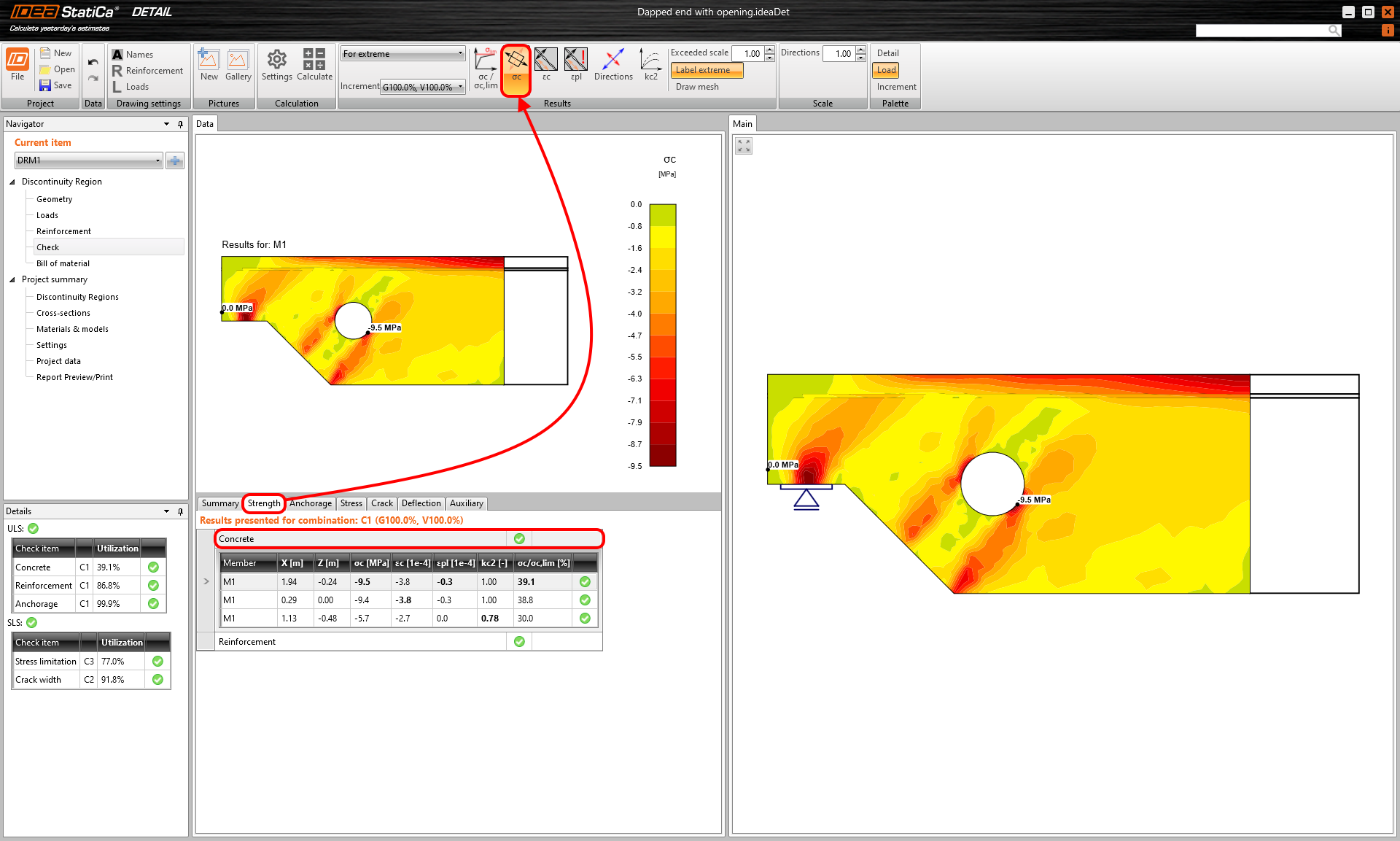Compressive concrete strainWhen you press the third icon in the result tab. The concrete strain εc for an applied portion of the load displays. Also known as principal strains ε2.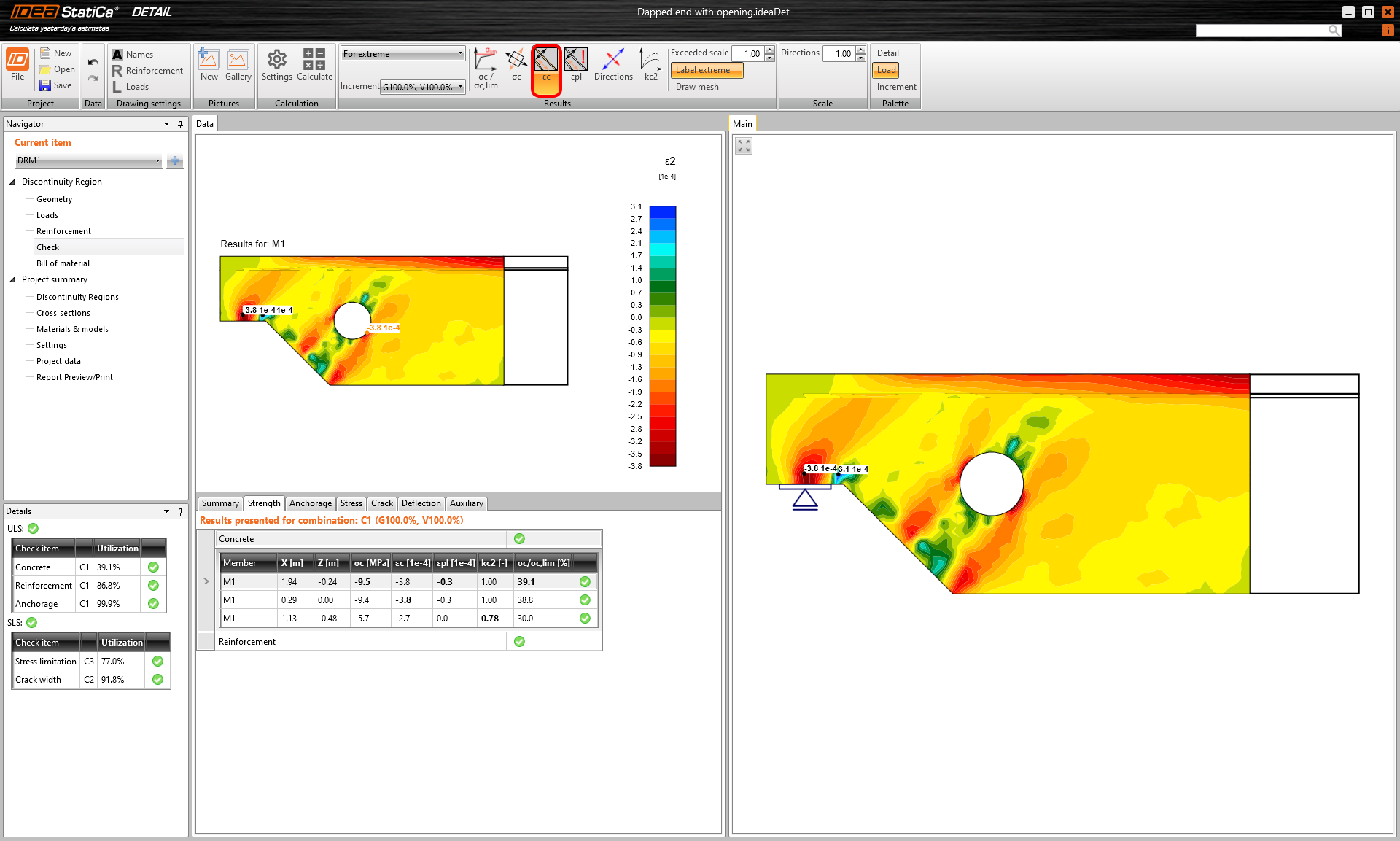Compressive plastic strain of concreteTo observe the occurrence of plastic behavior in the concrete, continue to the Compressive plastic strain of concrete. You can display the areas where the concrete is in the plastic branch of the stress-strain diagram.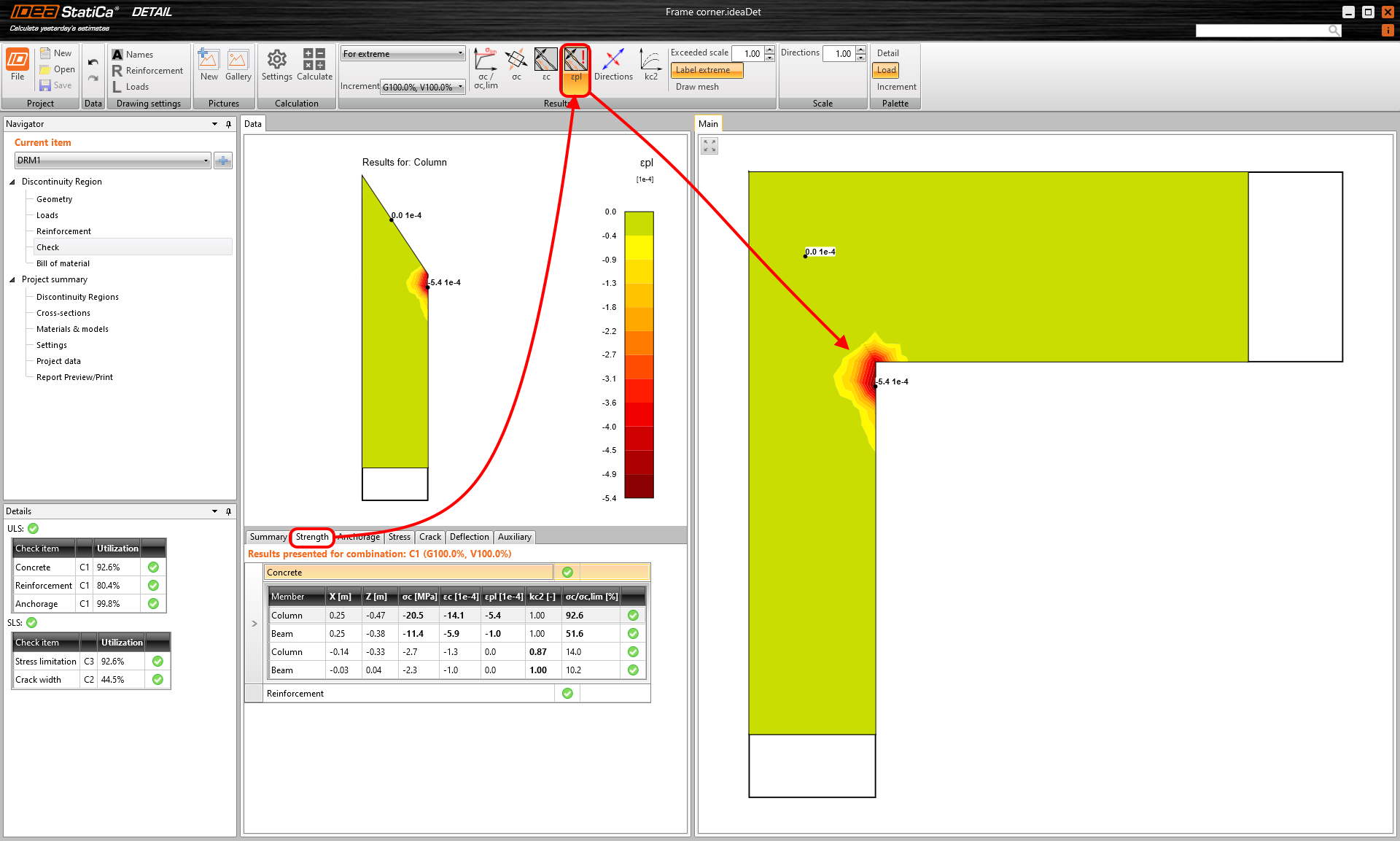Direction of principal stressesThis functionality shows the direction of principal stresses. As the tension in concrete is excluded, only the compressive vectors are displayed.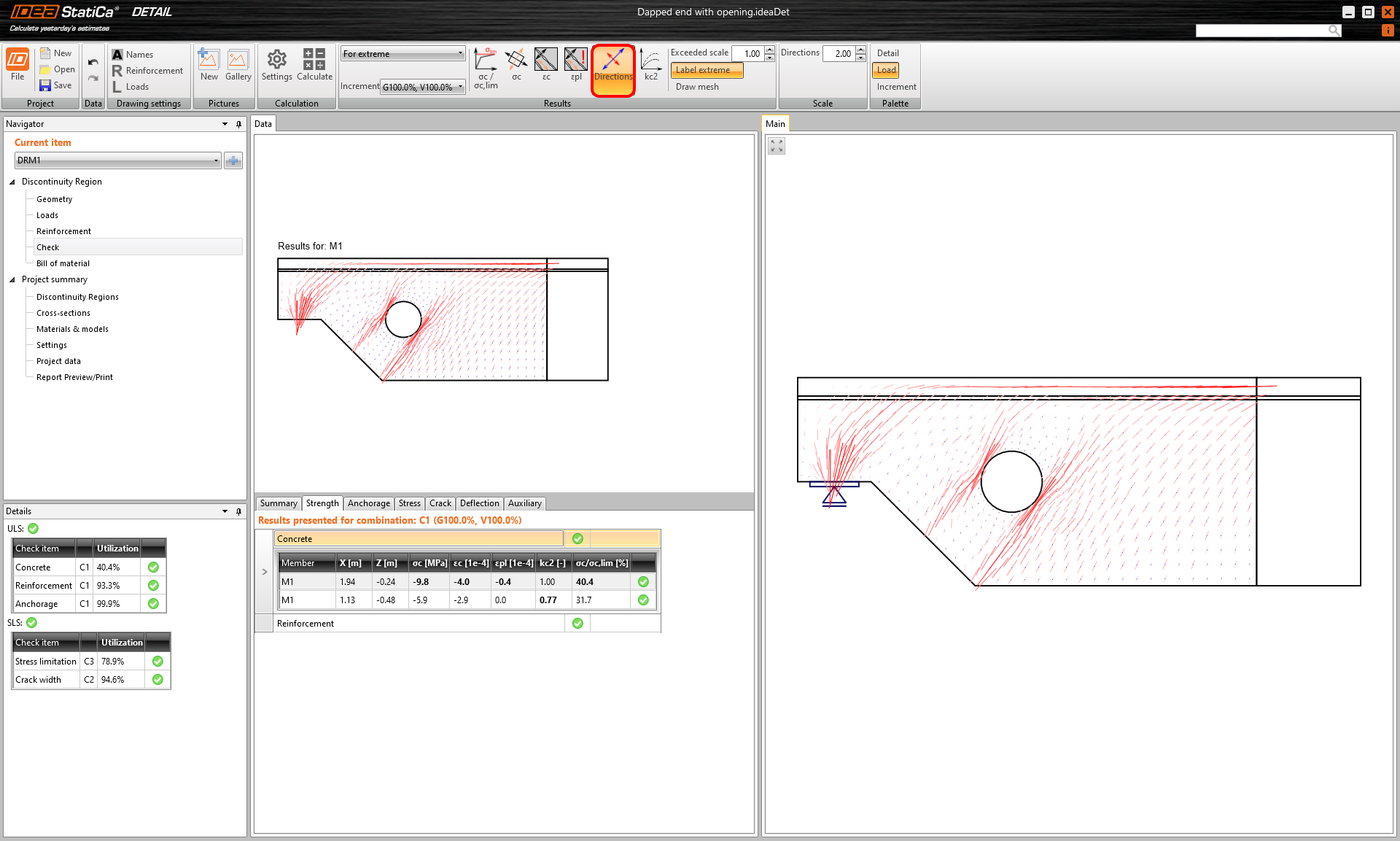Compressive strength reduction factorThe sixth option shows the distribution of the concrete compressive strength reduction due to transversal strain. This is the compression softening effect. This phenomenon (kc2), together with the increasing brittleness of concrete with strength, is taken into account through the factor kc.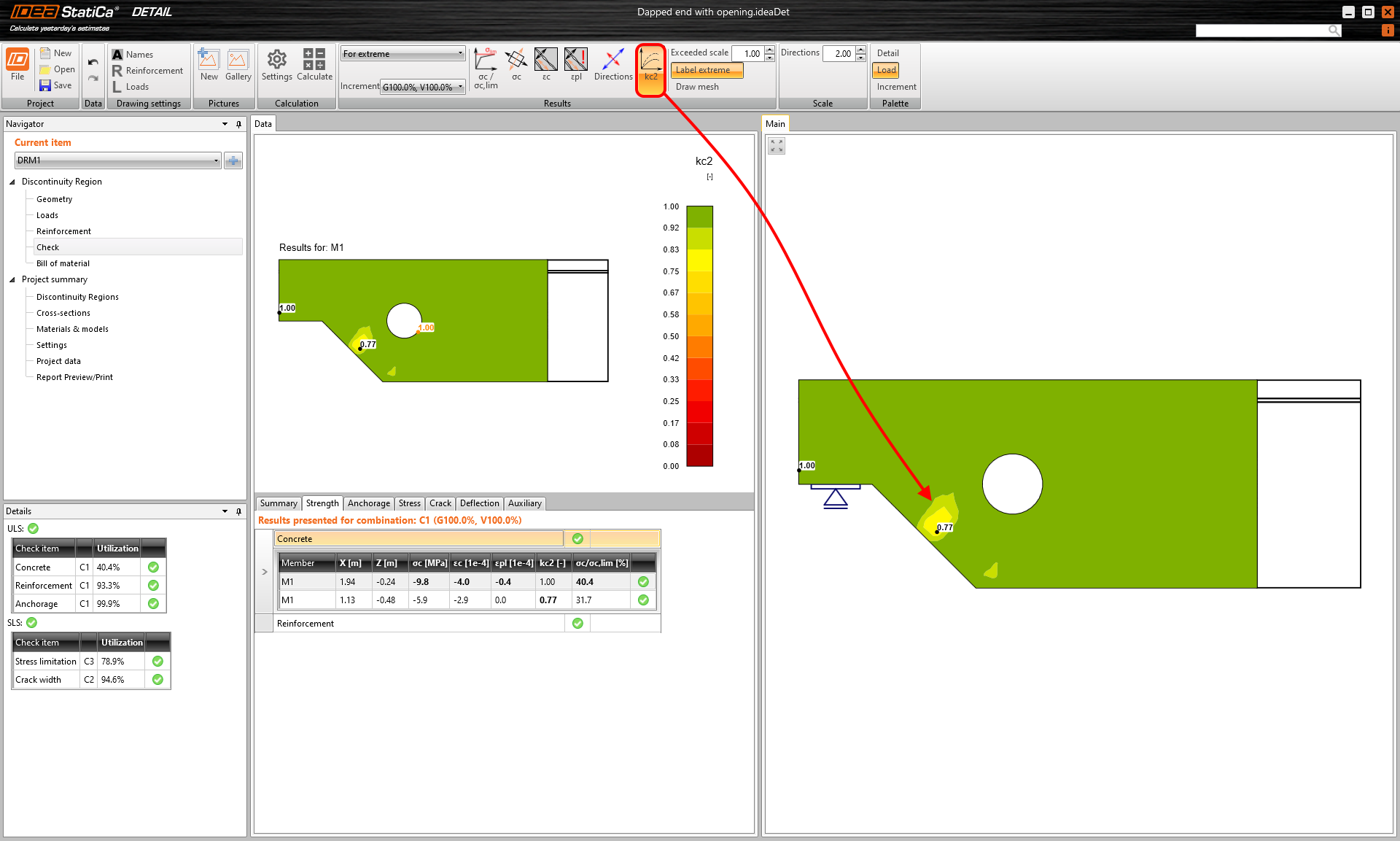To learn more, read the article: Material models (EN).ReinforcementFor steel, similar to concrete, we have more options for displaying the results. In general, it is possible to display stress and strain in reinforcement and the ratio of stress or strain and limit values.Ratio of stress or strain and limit valuesFirst two icons allow you to check the ratio of stress and strain to their limit values. The third icon shows a comparison of stress and yield stress. In other words, you can see the level of material utilization.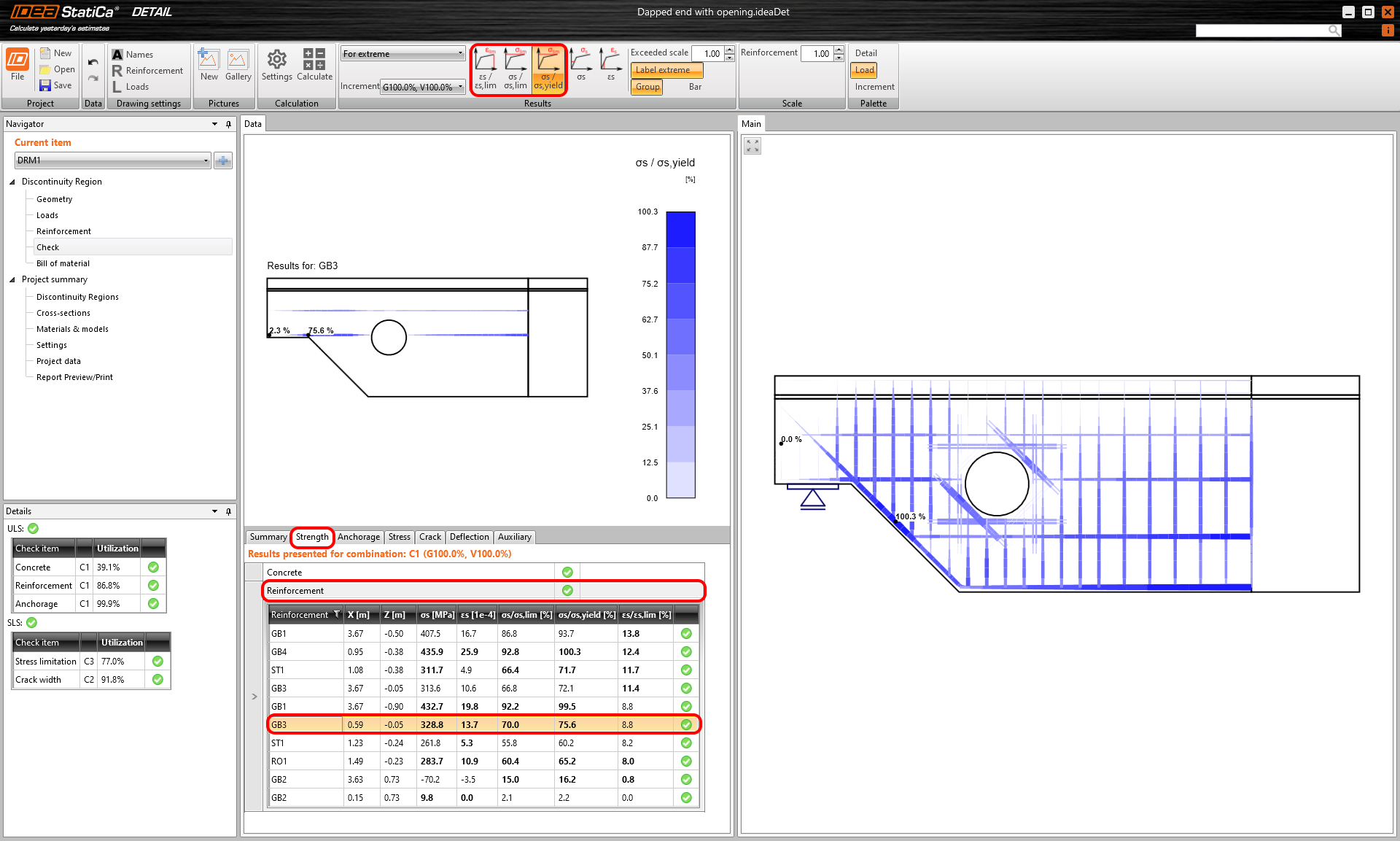Stress and strainIn addition to those mentioned above, there is an option to display the strain (εs) or the stress (σ2) of the reinforcement. In the data tab, you can observe the individual bars one by one. Or you can check the model and the overview in the Main window.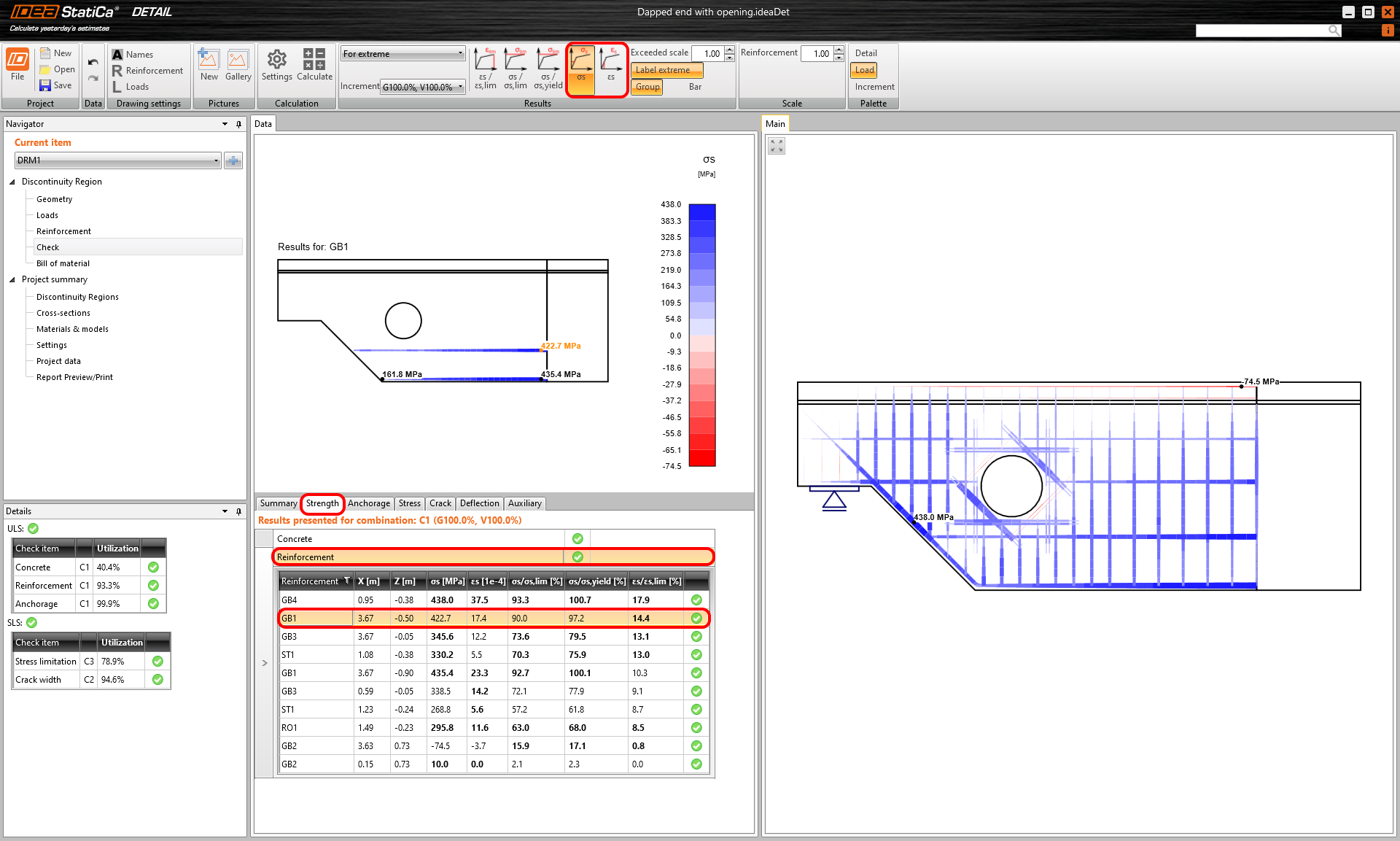AnchorageAnchorage are the last available results for ULS.To understand where the forces entering the calculation come from, let's discuss the individual components at first.Bond stress and bond forceThe bond stress 𝜏b (stress on the surface of the reinforcement bar) is calculated from the deformation of the bond element that represents the connection between concrete (2D element) and reinforcement (1D element). This relation is expressed by the stress-deformation function, see the figure below.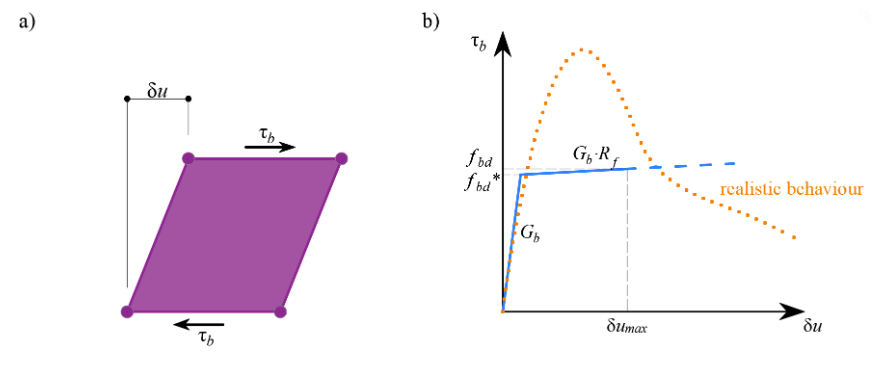Note that the slip is allowed.fbd* - yield bond stress fbd - limit bond stress Gb - shear stiffness in elastic part Rf - Coefficient for hardening δu, max - Ultimate slipOnce the plastic branch is reached - 𝜏b = fbd*, the stress (𝜏b) no longer increases so much compared to deformation (δu). At that point (𝜏b = fbd*), you get a bond stress ratio check (𝜏b/fbd) above 99 %. The check is passing until the limit deformation δu, max is reached.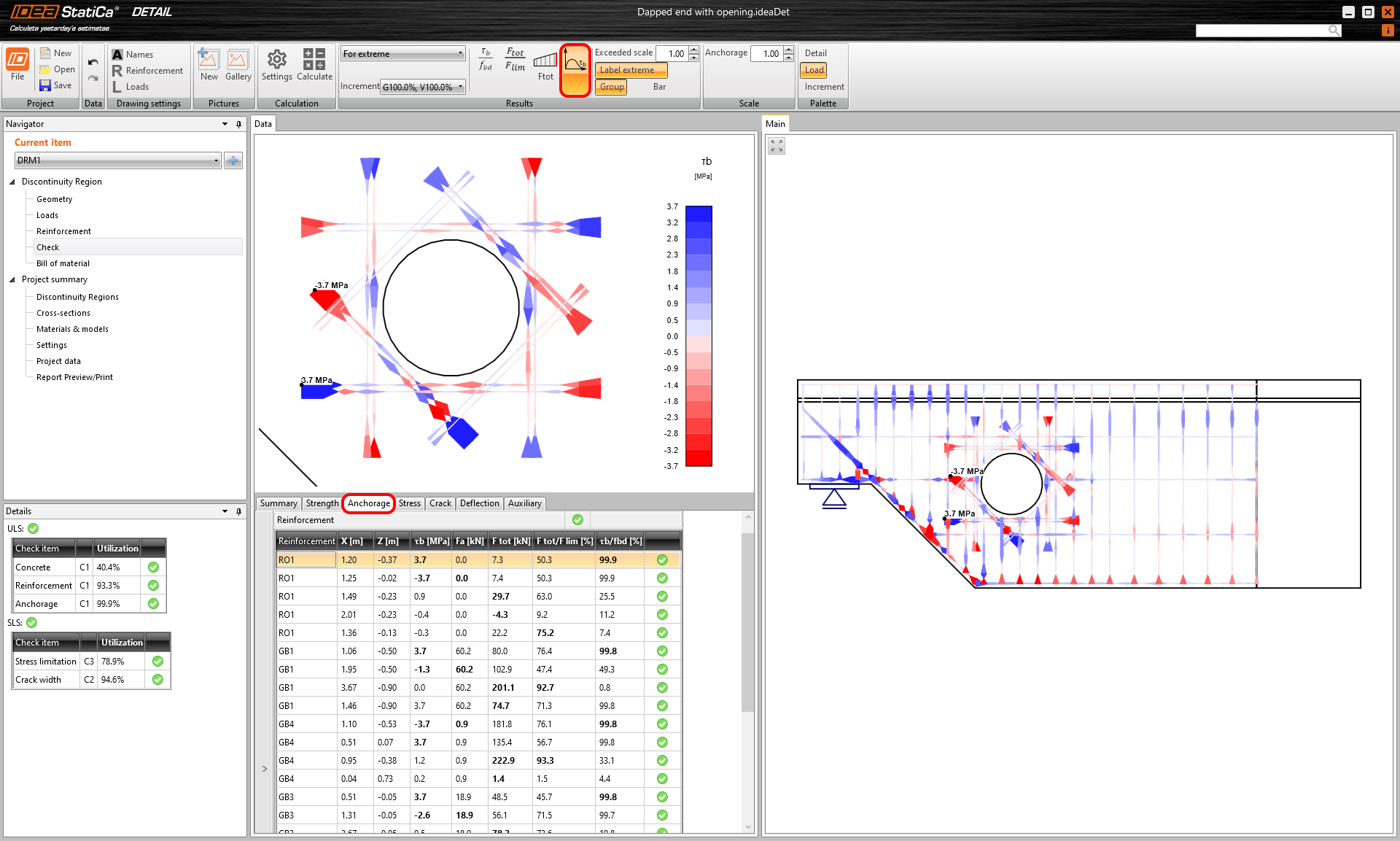By integrating the bond stress along each finite element of the reinforcement bar, you would get the bond force - Fbond for each bar element. The application does this integration for you.Anchorage force and total force in the barThe force transmitted by the spring that represents the selected anchorage type of reinforcement is called anchorage force. The maximum* anchorage force is calculated according to the following formula - Fa=β⋅As⋅fyd, ​where:β the anchorage coefficient based on anchorage type,As the cross-section of the reinforcing bar,fyd the design value of the yield strength of the reinforcement.*The maximum force can be achieved in the reinforcement at full utilization. In fact, the force transmitted by the spring will be calculated from the real stress in the reinforcement and will be further reduced by force trapped along the length of the reinforcement, depending on the bond model (cohesion) between concrete and reinforcement.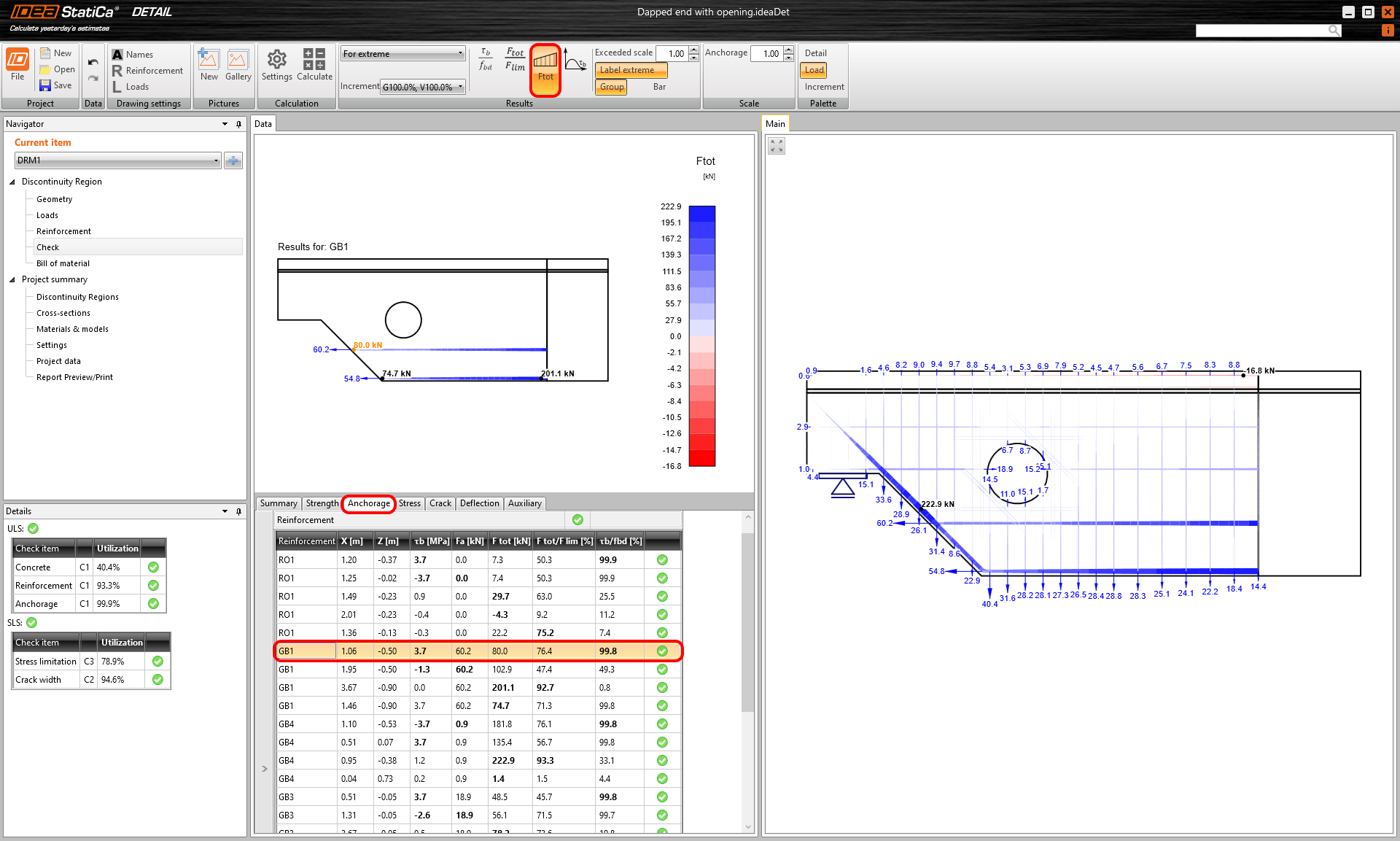Then the total force Ftot is basically the sum of the forces mentioned above, the anchorage force Fa and the bond force Fbond. The total force is equal to the force acting on the reinforcement from the load effects but spread over the entire length of the reinforcement. $F_{ext} = F_{tot}$$F_{tot} = F_a + F_{bond}$ To learn about more theory behind anchorage and to understand the bond model read the further article: Finite element types Bond stress check valueThe ratio of bond stress (𝜏b) and ultimate bond strength for selected (group of) bars is displayed. It shows the level of utilization with respect to ultimate bond strength between the rebar and adjacent concrete.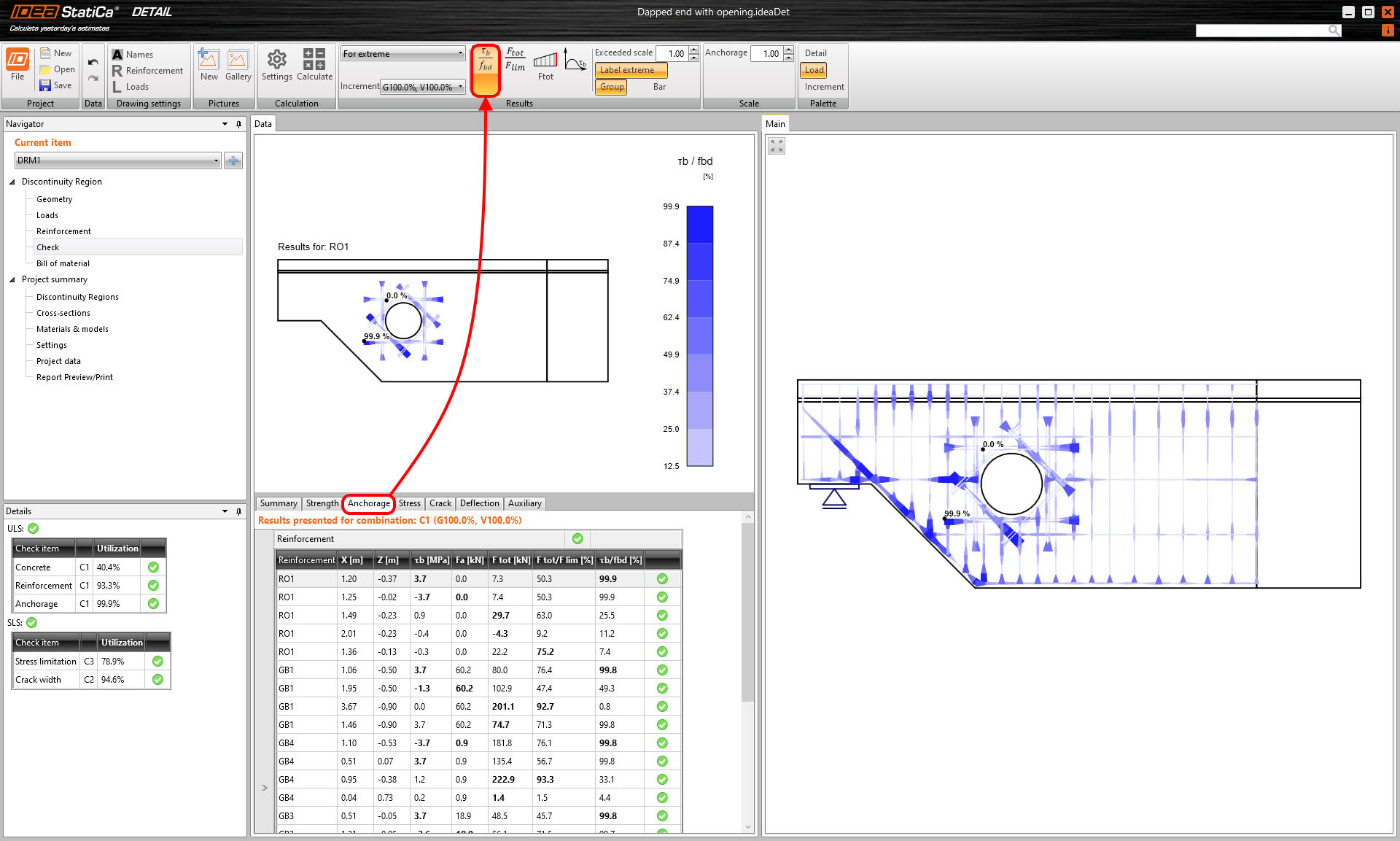Force check valueForce check value is the ratio of the total force (Ftot) in the bar and the limit value. The limit value of the force calculates as the minimum of two values: The force is calculated as the sum of the ultimate anchorage force and the force developed from the end of the bar to the point of interest, assuming the ultimate bond strength The ultimate strength of the bar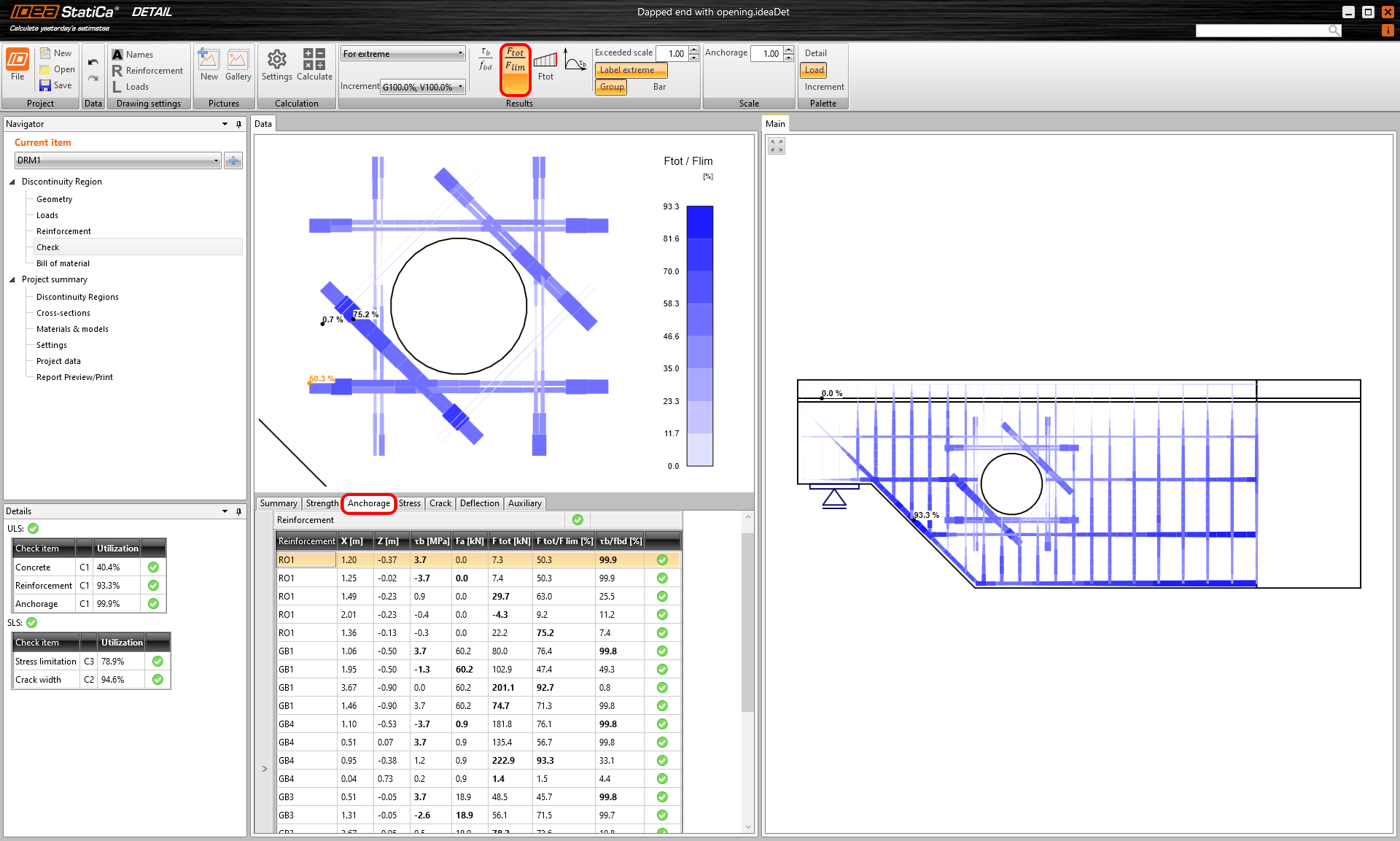In other words, Flim is the ultimate force in reinforcement, considering the ultimate anchorage force and ultimate resistance of the rebar.$$